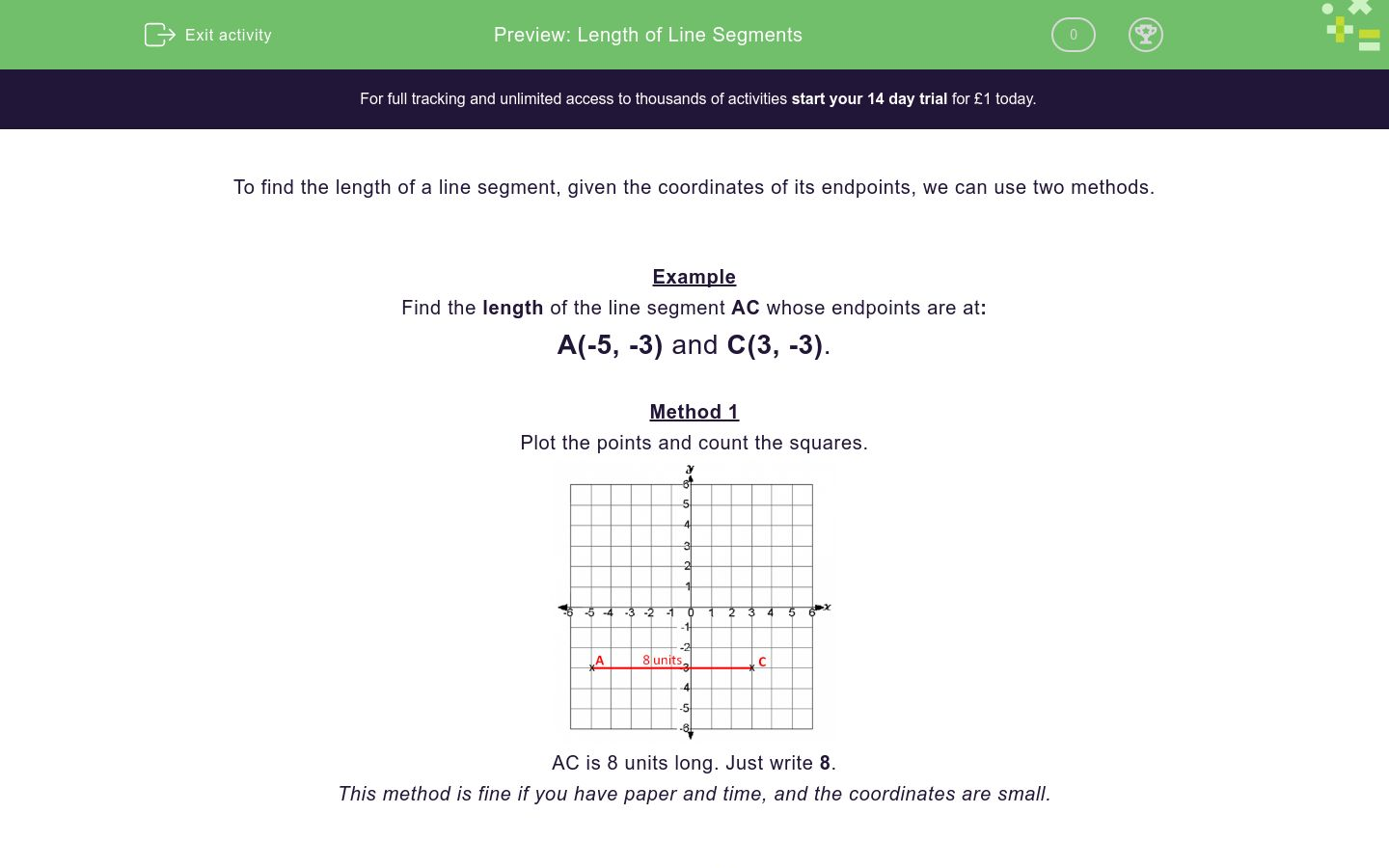# Length of Line Segments

In this worksheet, students must state the length of a vertical or horizontal line segment, given the coordinates of its endpoints.Key stage:  KS 3

Curriculum topic:  Algebra

Curriculum subtopic:  Use Coordinates in All Four Quadrants

Difficulty level:### QUESTION 1 of 10

To find the length of a line segment, given the coordinates of its endpoints, we can use two methods.

Example

Find the length of the line segment AC whose endpoints are at:

A(-5, -3) and C(3, -3).

Method 1

Plot the points and count the squares.AC is 8 units long. Just write 8.

This method is fine if you have paper and time, and the coordinates are small.

Method 2

Subtract the relevant coordinates.

The x-coordinates of the endpoints of vertical lines will be the same.

The y-coordinates of the endpoints of horizontal lines will be the same.

A is at (-5, -3) and C is at (3, -3).

The line AC is horizontal because the y-coordinates are both -3.

Subtract the x-coordinates:  -5 - 3 = -8 or 3 - -5 = 8.

Ignore the sign:  -8 or 8 means a length of 8 units. Just write 8.

For vertical lines, we subtract the y-coordinates.

Find the length of the line segment CD whose endpoints are at:

C(3, -3) and D(6, -3).

Find the length of the line segment FH whose endpoints are at:

F(-3, 3) and H(-3, 5).

Find the length of the line segment HI whose endpoints are at:

H(-3, 5) and I(-1, 5).

Find the length of the line segment FG whose endpoints are at:

F(-3, 3) and G(2, 3).

Find the length of the line segment EG whose endpoints are at:

E(2, -1) and G(2, 3).

Find the length of the line segment HJ whose endpoints are at:

H(-3, 5) and J(3, 5).

Find the length of the line segment AC whose endpoints are at:

A(-5, -3) and C(3, -3).

Find the length of the line segment AD whose endpoints are at:

A(-5, -3) and D(6, -3).

Find the length of the line segment JC whose endpoints are at:

J(3, 5) and C(3, -3).

Find the length of the line segment AB whose endpoints are at:

A(-5, -3) and B(-1, -3).

• Question 1

Find the length of the line segment CD whose endpoints are at:

C(3, -3) and D(6, -3).

3
EDDIE SAYS
Method 1: Count squares on the diagram between C and D.Method 2: 6 - 3 = 3
• Question 2

Find the length of the line segment FH whose endpoints are at:

F(-3, 3) and H(-3, 5).

2
EDDIE SAYS
Method 1: Count squares on the diagram between F and H.Method 2: 5 - 3 = 2
• Question 3

Find the length of the line segment HI whose endpoints are at:

H(-3, 5) and I(-1, 5).

2
EDDIE SAYS
Method 1: Count squares on the diagram between H and I.Method 2: -1 - -3 = -1 + 3 = 2
• Question 4

Find the length of the line segment FG whose endpoints are at:

F(-3, 3) and G(2, 3).

5
EDDIE SAYS
Method 1: Count squares on the diagram between F and G.Method 2: 2 - -3 = 2 + 3 = 5
• Question 5

Find the length of the line segment EG whose endpoints are at:

E(2, -1) and G(2, 3).

4
EDDIE SAYS
Method 1: Count squares on the diagram between E and G.Method 2: 3 - -1 = 3 + 1 = 4
• Question 6

Find the length of the line segment HJ whose endpoints are at:

H(-3, 5) and J(3, 5).

6
EDDIE SAYS
Method 1: Count squares on the diagram between H and J.Method 2: 3 - -3 = 3 + 3 = 6
• Question 7

Find the length of the line segment AC whose endpoints are at:

A(-5, -3) and C(3, -3).

8
EDDIE SAYS
Method 1: Count squares on the diagram between A and C.Method 2: 3 - -5 = 3 + 5 = 8
• Question 8

Find the length of the line segment AD whose endpoints are at:

A(-5, -3) and D(6, -3).

11
EDDIE SAYS
Method 1: Count squares on the diagram between A and D.Method 2: 6 - -5 = 6 + 5 = 11
• Question 9

Find the length of the line segment JC whose endpoints are at:

J(3, 5) and C(3, -3).

8
EDDIE SAYS
Method 1: Count squares on the diagram between J and C.Method 2: 5 - -3 = 5 + 3 = 8
• Question 10

Find the length of the line segment AB whose endpoints are at:

A(-5, -3) and B(-1, -3).

4
EDDIE SAYS
Method 1: Count squares on the diagram between A and B.Method 2: -1 - -5 = -1 + 5 = 4
---- OR ----

Sign up for a £1 trial so you can track and measure your child's progress on this activity.

### What is EdPlace?

We're your National Curriculum aligned online education content provider helping each child succeed in English, maths and science from year 1 to GCSE. With an EdPlace account you’ll be able to track and measure progress, helping each child achieve their best. We build confidence and attainment by personalising each child’s learning at a level that suits them.

Start your £1 trial